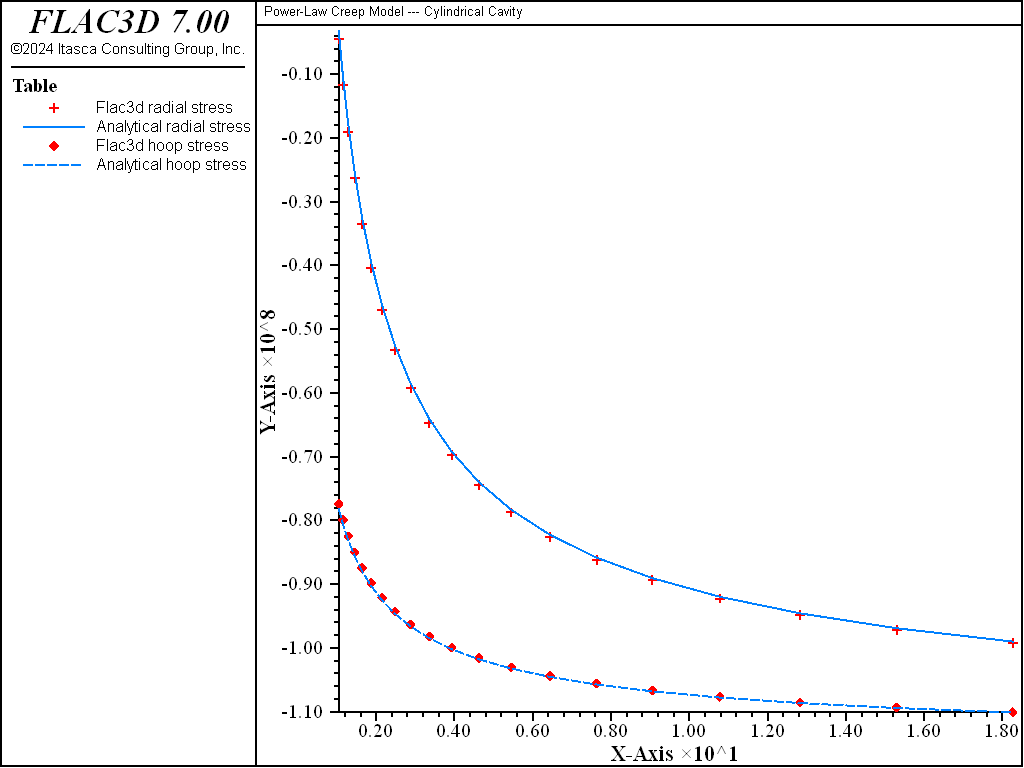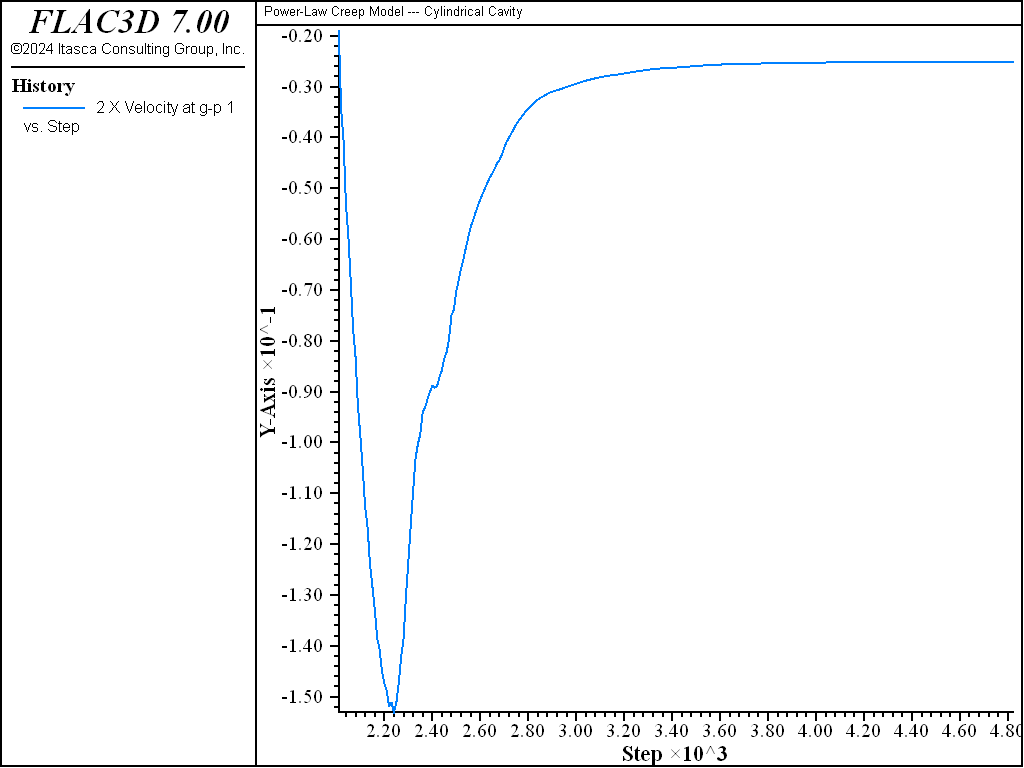# Power Model: Cylindrical Cavity

Note

To view this project in FLAC3D, use the menu command Help ► Examples…. Choose “Creep/CylindricalCavityPower” and select “CylindricalCavityPower.prj” to load. The project’s main data files are shown at the end of this example.

The power-law creep model in FLAC3D is used to solve the problem of radial creep of an infinitely long, thick-walled cylinder. A comparison is made with an analytical solution.

The cylinder is subject to a pressure on the outer surface. The creep behavior of the material is defined by a single-component power law:

$\dot \epsilon_{cr} = A \bar \sigma^n$

For this problem, $$A$$ = 1 × 10-7 MPa-3 yr-1 (or 1 × 10-25 Pa-3 yr-1), and $$n$$ = 3. The elastic properties of the material are $$E$$ = 820 MPa and $$\nu$$ = 0.3636.

An analytical steady-state solution to this problem has been provided by van Sambeek (1986) and is reproduced here:

$\begin{split}\begin{split} \sigma_r &= - P_b + P_b\ \biggl[ {{(b/r)^{2/n} - 1} \over {\ (b/a)^{2/n}} - 1} \biggr] \\ \sigma_\theta &= - P_b - P_b \biggl[ {{[(2-n)/n]\ (b/r)^{2/n} + 1} \over {(b/a)^{2/n}} - 1} \biggr] \\ \sigma_z &= - P_b - P_b \biggl[ {{[(1-n)/n]\ (b/r)^{2/n} + 1} \over {(b/a)^{2/n}} - 1} \biggr] \\ \dot u_r &= - A\ {(3/4)^{(n+1)/2}} \ {\biggl[ P_b\ {{2/n} \over {(b/a)^{2/n} - 1}} \biggr]}^n {b^2/r} \end{split}\end{split}$

where: $$\sigma_r$$, $$\sigma_\theta$$, are radial and tangential stress components;

 $$\sigma_z$$ is the out-of-plane stress component; $$P_b$$ is the applied boundary stress; $$\dot u_r$$ is the radial displacement rate; $$a$$, $$b$$ are the inner and outer radii of the cylinder, respectively; and $$r$$ is the radius to point of calculation.

Figure 1 shows the FLAC3D grid. Taking advantage of the axial symmetry, only a sector of 9° is represented. A single layer of zones is used in the out-of-plane direction. The inner radius is $$a$$ = 1 and the outer radius is $$b$$ = 20.

Gridpoints were fixed in the out-of-plane direction and in the circumferential direction. A pressure of 100 MPa was applied at the outer boundary.

The initial stresses, corresponding to an elastic cylinder in plane strain without a hole, were

$\sigma_{xx} = \sigma_{yy} = \sigma_{zz} = -P_b = -100 {\hbox{ MPa}}$

The cylinder was allowed to come to elastic equilibrium by setting creep to off. Then, the creep timestep was set to its initial value (defined by model creep timestep minimum) and allowed to increase automatically until steady state was reached. The maximum allowable timestep (defined by model creep timestep maximum) was determined by the procedure described in Solving Creep Problems.

The data file for this problem is given at the end of this section. The analytical solution is included in the project as a FISH function powcyl.

The results of this example are summarized in Figure 2 through Figure 5. Figure 2 compares the analytical solution for the radial velocity in the steady-state condition with the FLAC3D results. Figure 3 shows the comparison of radial and hoop stresses. Figure 4 shows the history of the radial velocity at the cavity. Some oscillation occurs initially, but the steady-state solution is quickly reached. The initial high value is to be expected since the pre-creep state is far from equilibrium. The final value is within 2.5% of the analytical solution. Figure 5 shows the evolution of the timestep from its initial value of 10-4 to the maximum value of 1.Figure 3: Comparison of radial and hoop stress at steady state—FLAC3D radial stress versus analytical radial stress and FLAC3D hoop stress versus analytical hoop stress.Figure 4: History of radial velocity at the cylindrical cavity.

Data File

;------------------------------------------------------------
;       cylindrical cavity -- power law model
;-------------------------------------------------------------------
model new
model large-strain off
fish automatic-create off
model title "Power-Law Creep Model --- Cylindrical Cavity"
model configure creep
;
zone create brick  point 0 1 0 0               point 1 20 0 0           ...
point 3 0.98769 0.15643 0   point 6 19.754 3.129 0   ...
point 2 1 0 -1              point 4 20 0 -1          ...
point 5 0.98769 0.15643 -1  point 7 19.754 3.129 -1  ...
size 20 1 1  ratio 1.2 1 1
zone face skin
zone cmodel power
; Properties and stresses in Pascal units (not MPa)
zone property bulk=1e9 shear=3e8 constant-1=1e-25 exponent-1=3
;
zone gridpoint fix velocity-y range position-y 0
zone gridpoint fix velocity-z range position-y 0
zone face apply velocity-normal 0 range group 'North'
zone face apply velocity-dip    0 range group 'North'
zone face apply stress-normal -100e6 range group 'East'
zone initialize stress xx -100e6 yy -100e6 zz -100e6
;
model step 2000
model save 'cyl_1'
;
model history mechanical unbalanced-maximum
zone history velocity-x gridpointid 1
zone history velocity-x gridpointid 81
zone history displacement-x gridpointid 1
zone history displacement-x gridpointid 81
zone history stress-xx gridpointid 1
zone history stress-yy gridpointid 1
zone history stress-zz gridpointid 1
model history creep time-total
model history timestep
;
zone gridpoint initialize velocity (0,0,0)
model creep active on
model creep timestep starting 1.0e-4
model creep timestep minimum 1.0e-4
model creep timestep maximum 1.0
model solve time-total 2000
model save 'cyl_2'
; compare analytic solution......
program call 'solution' suppress
table  '1' label 'Flac3d x-velocity'
table '11' label 'Analytical x-velocity'
table  '2' label 'Flac3d radial stress'
table '12' label 'Analytical radial stress'
table  '3' label 'Flac3d hoop stress'
table '13' label 'Analytical hoop stress'
table  '4' label 'Flac3d out-of-plane stress'
table '14' label 'Analytical out-of-plane stress'
model save 'cylindrical-cavity-power'